# unwrapMultipart

Unwrap angles with parts separated by `NaN` values

## Syntax

``Q = unwrapMultipart(P)``
``Q = unwrapMultipart(P,angleUnit)``

## Description

example

````Q = unwrapMultipart(P)` unwraps the azimuths, longitudes, or phase angles in vector `P`. This syntax assumes the angle units are in radians. Whenever the jump between consecutive angles is greater than or equal to π radians, the function shifts the angles by adding multiples of ±2π until the jump is less than π. When `P` includes multiple parts separated by `NaN` values, the function unwraps each part independently. When `P` has only one part, the result is equivalent to `unwrap(P)`. ```

example

````Q = unwrapMultipart(P,angleUnit)` specifies the angle units `angleUnit` of the angles in `P`. When `P` contains angles in degrees, specify `angleUnit` as `"degrees"`. Whenever the jump between consecutive angles is greater than or equal to 180 degrees, this syntax shifts the angles by adding multiples of ±360 until the jump is less than 180. The default for `angleUnit` is `"radians"`, which specifies the units as radians. ```

## Examples

collapse all

Create a vector of phase angles from 0 to 4$\pi$ that is separated into two parts by a `NaN` value. Use the phase angles to define the $\mathit{xy}$-coordinates of a spiral.

```t1 = linspace(0,1.75*pi); t2 = linspace(2.25*pi,4*pi); t = [t1 NaN t2]; x = t/pi.*cos(t); y = t/pi.*sin(t); plot(x,y)```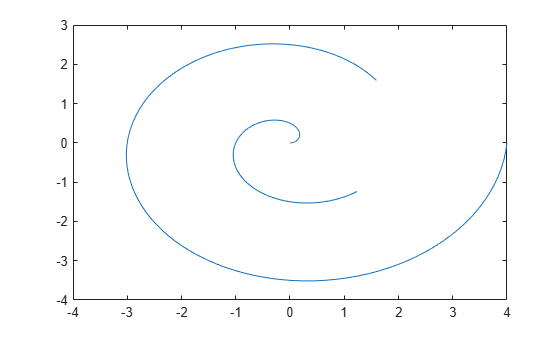Find the phase angle of the spiral from the $\mathit{xy}$-coordinates by using the `atan2` function. The `atan2` function returns the angle values within the closed interval from $-\pi$ to $\pi$.

```P = atan2(y,x); plot(t,P)```Note that the plot has discontinuities. Eliminate the discontinuities by using the `unwrapMultipart` function. The `unwrapMultipart` function independently unwraps the `NaN`-separated parts of the vector.

```Q1 = unwrapMultipart(P); plot(t,Q1)```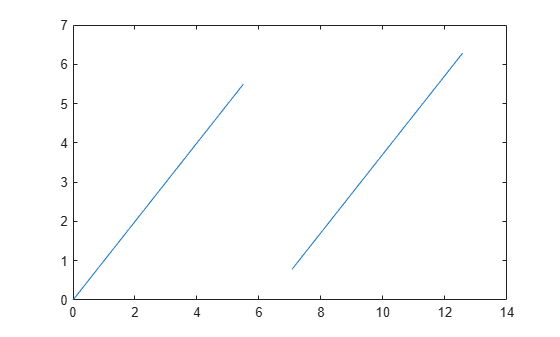Compare the result with the `unwrap` function, which does not independently unwrap `NaN`-separated parts.

```Q2 = unwrap(P); plot(t,Q2)```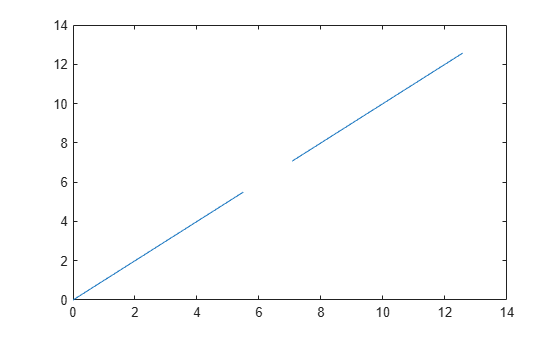Create a vector of longitudes from 0 to 720 degrees that is separated into two parts by a `NaN` value.

`lon = [0:20:340 NaN 380:20:720];`

Wrap the longitudes to the closed interval from –180 to 180 degrees by using the `wrapTo180` function.

```P = wrapTo180(lon); plot(P)```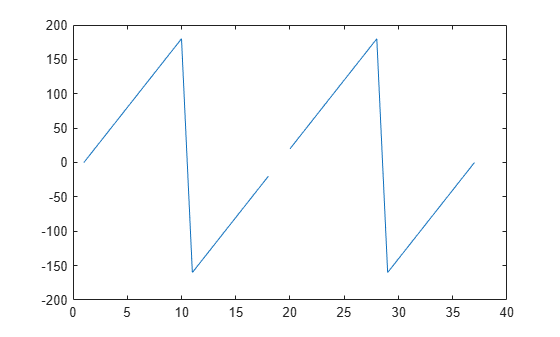Unwrap the longitude angles by using the `unwrapMultipart` function. The function independently unwraps the `NaN`-separated parts of the vector.

```Q = unwrapMultipart(P,"degrees"); plot(Q)```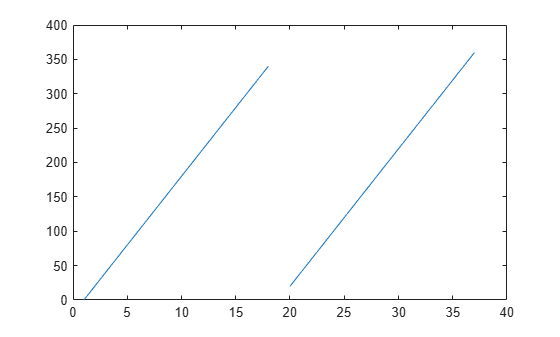Compare the result to the `unwrap` function, which works only in radians and does not independently unwrap `NaN`-separated parts.

```rad = deg2rad(P); Q2 = unwrap(rad); plot(Q2)```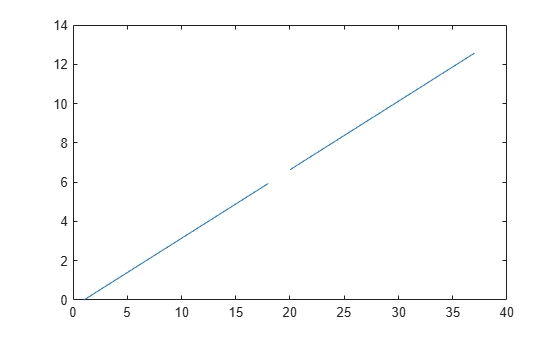## Input Arguments

collapse all

Input angles, specified as a row or column vector.

Angle unit, specified as one of these options:

• `"degrees"` — Degrees

• `"radians"` — Radians

Data Types: `char` | `string`

## Output Arguments

collapse all

Unwrapped angles, returned as a vector of the same size as `P`. The `NaN` values in `Q` correspond to the `NaN` values in `P`.

## Version History

Introduced in R2007b